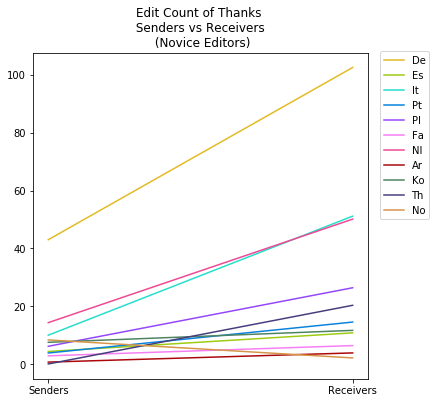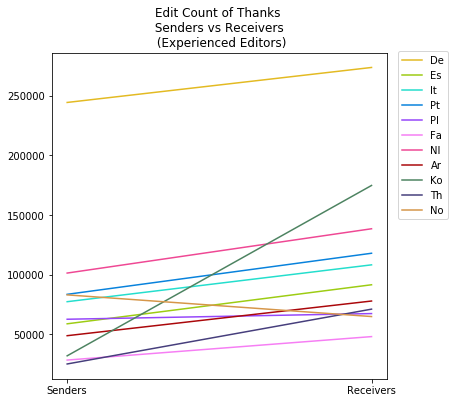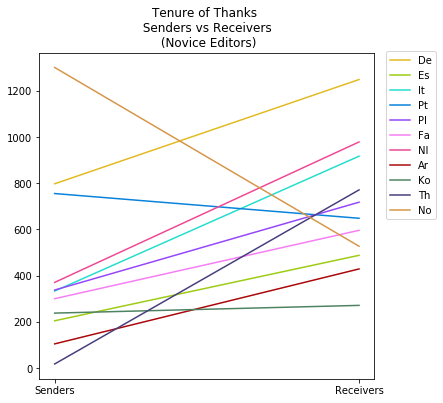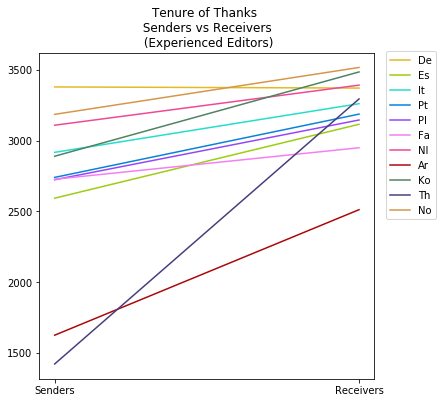#### Introduction¶

This notebook contains a study of the average characteristics of thanks senders vs thanks receivers. The goal is to characterize both populations by features such as edit count and tenure (number of days since registration).

import csv
from datetime import datetime


#### SQL Query¶

• Takes every person who has received a thank in some month and finds the number of thanks they received as well as their total edit count. Orders them from smallest to largest by edit count. (Can also use this query structure to get thanks they sent).

use PROJECT;

select A.user_name as Username, A.num_edits as Edits, A.registration as Registration, B.num_thanks as Thanks

from (select user_name, user_editcount as num_edits, user_registration as registration from user) as A

join (select log_title, count(log_title) as num_thanks from logging_userindex where log_action = 'thank' and log_type='thanks' and log_timestamp >= timestamp('2018-05-01') and log_timestamp < timestamp('2018-06-01') group by log_title limit 5000) as B

on A.user_name = B.log_title

order by A.num_edits;

def ints_to_datetime(year, month, day):
return datetime(year, month, day)

#information for converting date strings to a consistent format
snapshot = ints_to_datetime(2018, 6, 1)
registration_intro_date = ints_to_datetime(2009, 2, 7)
dtime_format = "%Y%m%d"
dtime_len = 8

#define filenames
src = '(1-3)-data/'
languages = ['De', 'Es', 'It', 'Pt', 'Pl', 'Fa', 'Nl', 'Ar', 'Ko', 'Th', 'No']
input_suffixes = ['Senders.csv', 'Receivers.csv']
output_suffixes = ['NoviceAvgs.csv', 'ExperiencedAvgs.csv']
samples = [[0, 0.2], [0.8, 1]]

def make_files_lst(suffixes, src=src, languages=languages):
files_lst = []
for language in languages:
lst = []
for suffix in suffixes:
lst.append(src+language+suffix)
files_lst.append(lst)
return files_lst

input_files = make_files_lst(input_suffixes)

output_files = make_files_lst(output_suffixes)

def str_to_datetime(strtime, dtime_format=dtime_format, dtime_len=dtime_len):
return datetime.strptime(strtime[:dtime_len], dtime_format)

#subtracts two datetimes to give a number of days
def tenure_length(d1, d2=snapshot):
return abs((d2-d1).days)

#take the data from the sql queries and reformat it
def make_senders_vs_receivers_csvs(input_files, output_files, samples):
#use the sender / receiver edits data to compare different samplings of senders and receivers
for i in range(0, len(samples)):
senders_avg = find_sample_avg(input_files, samples[i])
receivers_avg = find_sample_avg(input_files, samples[i])
with open(output_files[i], 'w') as csvfile:
fieldnames = ['Senders Edits', 'Receivers Edits', 'Senders Tenure', 'Receivers Tenure']
wrter = csv.DictWriter(csvfile, fieldnames=fieldnames)

#find the average edit count of all editors within a sample
#a sample is some percentage of editors (ex top 20) based on their edit count
def find_sample_avg(input_file, sample):
#return some sampling of the input_file
with open(input_file, 'r', encoding = 'utf-8') as csvfile:
file_size = 0
for row in rder:
file_size += 1
start_row = int(file_size * sample)
end_row = int(file_size * sample)

with open(input_file, 'r', encoding = 'utf-8') as csvfile:
i = 0
edits_sum = 0
tenure_sum = 0
num_thanks = 0
for row in rder:
if (start_row <= i and i < end_row):
thanks = int(row['Thanks'])
num_thanks += thanks
edits_sum += int(row['Edits']) * thanks #count each editor n times, n = their thanks
if (row['Registration'] == ''):
d1 = registration_intro_date
else:
d1 = str_to_datetime(row['Registration'])
tenure_sum += tenure_length(d1) * thanks
i+=1
sravg_thanks = (edits_sum * 1.0) / num_thanks
sravg_tenure = (tenure_sum * 1.0) / num_thanks
return [sravg_thanks, sravg_tenure]

def format_data(samples=samples, input_files=input_files, output_files=output_files):
for i in range(0, len(input_files)):

#format_data()

#pretty print data
def output_data(input_files=output_files):
headers = ['Language Sample', 'Senders Edits', 'Receivers Edits',
'Senders Tenure', 'Receivers Tenure']
for language_files in output_files:
for fle in language_files:
with open(fle, 'r', encoding='utf-8') as csvfile:
for row in rder:
row['Language Sample'] = fle
print(row)

#output_data(output_files)

import numpy as np
import matplotlib.pyplot as plt

#make names for figure files
filenames = ['sr-novice-edits', 'sr-experienced-edits', 'sr-novice-tenure', 'sr-experienced-tenure']
for i, filename in enumerate(filenames):
filenames[i] = '../figures/' + filename + '.png'

colors = ['#E3BA22', '#9DCC13', '#24DECD', '#0882DC', '#9546FA', '#F67EF4', '#EF4894', '#AA070A', '#4D8361', '#463E7C', '#D69649']

def make_plot(title, filename, input_files=output_files, experience_level=0, comparison='edits', colors=colors):
xaxis = ['Senders', 'Receivers']
plt.rcParams["figure.figsize"] = [6, 6]
fig,ax = plt.subplots()
for i in range(0, len(input_files)):
language_files = input_files[i]
fle = language_files[experience_level]
if (comparison == 'edits'):
fieldnames = ['Senders Edits', 'Receivers Edits']
else:
fieldnames = ['Senders Tenure', 'Receivers Tenure']
with open(fle, 'r', encoding='utf-8') as csvfile:
yaxis = []
for row in rder:
yaxis = [float(row[k]) for k in fieldnames]
#ax.set_yticklabels([]) -- would hide markings
ax.plot(xaxis, yaxis, colors[i])
ax.legend(languages, loc='upper left', bbox_to_anchor=(1.02, 1.02))
plt.title(title)
plt.savefig(filename, bbox_inches='tight')
plt.show()

make_plot('Edit Count of Thanks \n Senders vs Receivers \n (Novice Editors)', filenames)make_plot('Edit Count of Thanks \n Senders vs Receivers \n (Experienced Editors)', filenames, experience_level=1)make_plot('Tenure of Thanks \n Senders vs Receivers \n (Novice Editors)', filenames, experience_level=0, comparison='tenure')make_plot('Tenure of Thanks \n Senders vs Receivers \n (Experienced Editors)', filenames, experience_level=1, comparison='tenure')#### Conclusion¶

Thanks are on average sent "upwards", or to more experienced editors. This is true both when looking at the sample of the bottom 20% of editors (in terms of edit count) and the sample of the top 20%, and the only language studied which consistently broke this mold was Norweigian (No), which was perhaps tellingly the smallest language we studied (by monthly active editor count.